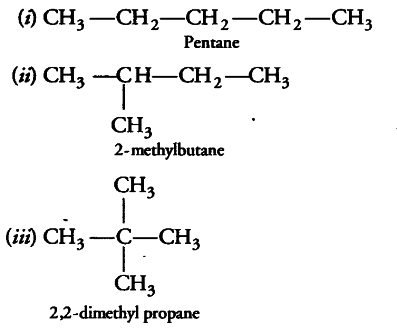# An alkane has a molecular mass of 72

An alkane has a molecular mass of 72. Draw all its possible chain iosmers and write their IUPAC names

First of all, we will derive the molecular formula.
Moleccular formula of alkane is { C }_{ n } H2n+2
Molecular mass = 72
.’. 12n+2n+2 = 72
n= 5
The alkane is C_{5}H_{12}.The possible chain isomers are